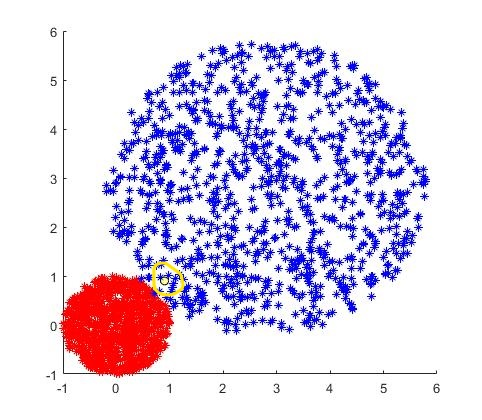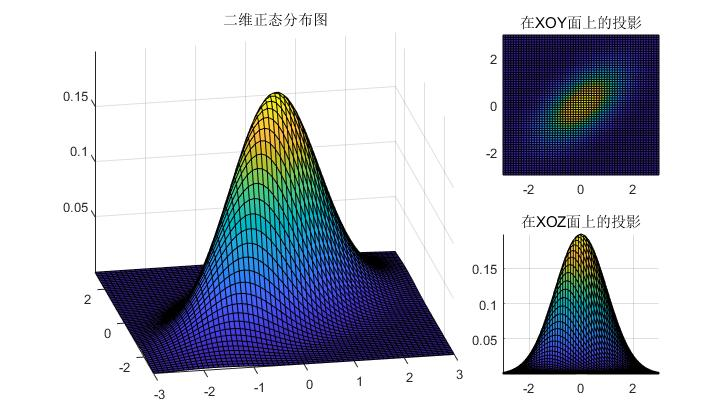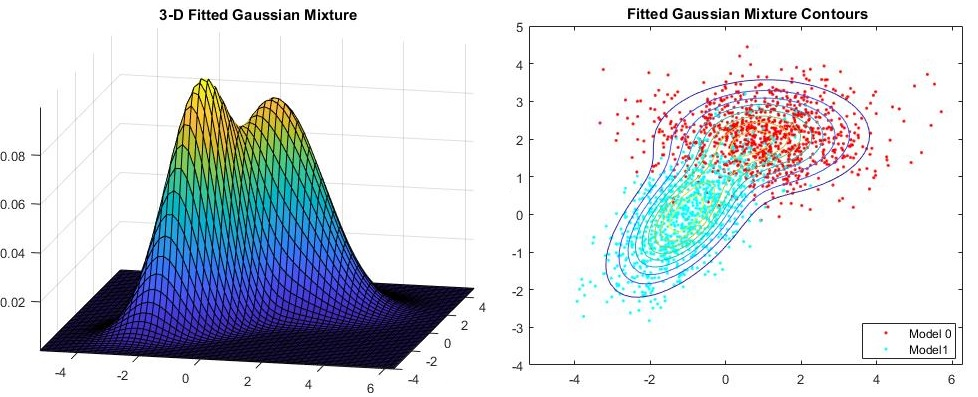## 高斯分布：

\begin{aligned} &p(x)=\frac{1}{\sqrt{2}\pi}exp(-\frac{x^2}{2}) \ &p(x,y)=p(x)*p(y)=\frac{1}{\sqrt{2}\pi}exp(-\frac{x^2+y^2}{2}) \end{aligned}

$$p(v) = \frac{|A|}{\sqrt{2}\pi}exp[-\frac{1}{2}(x-\mu)\Sigma^{-1}(x-\mu)]$$## 高斯混合分布：

$$p(x)=\sum_{k=1}^{K}\pi_{k}\mathcal{N}(x|\mu_{k},\Sigma_K)$$## 高斯混合分布求解：

### 初级求解方式：

$$f=\prod_{i=1}^N p(x_i)$$

$$max\Sigma_{i=1}^{N}log(\Sigma_{k=1}^{K}\pi_k\mathcal{N}(x_i|\mu_k,\sigma_k))$$

$$\omega_i(k)=\frac{\pi_k\mathcal{N}(x|\mu_k,\sigma_k)}{\Sigma_{j=1}^{K}\sigma_j\mathcal{N}(x|\mu_j,\sigma_j)}$$

\begin{aligned} & \mu_k=\frac{1}{N}\Sigma_{i=1}^{N}\omega_i(k)x_i \ & \sigma_k=\frac{1}{N}\Sigma_{i=1}^{N}\omega_i(k)(x_i-\mu_k)(x_i-\mu_k)^T \ &N_k=\Sigma_{i=1}^{N}\omega_i(k) \end{aligned}

### 类别数目未知的情况：

$$E(x)=f(x)+r$$

\begin{aligned} E(x)&=p_t(x)-p(x)+r\ &=p_t(x)-\sum_{k=1}^{K}\pi_{k}\mathcal{N}(x|\mu_{k},\Sigma_K) \end{aligned}+\alpha\sum_{i}^K|\pi_i|